Click to Chat

1800-1023-196

+91-120-4616500

CART 0

• 0

MY CART (5)

Use Coupon: CART20 and get 20% off on all online Study Material

ITEM
DETAILS
MRP
DISCOUNT
FINAL PRICE
Total Price: Rs.

There are no items in this cart.
Continue Shopping• Complete Physics Course - Class 11
• OFFERED PRICE: Rs. 2,968
• View Details

```Refraction

Introductory Concepts

Refraction

Refractive Index

Snell’s Law or Laws of Refraction

Simple Application of Refraction

Refraction through Lens

Concave Lens

Convex Lens

Image formation by Lenses

Image formation by Convex Lens

Image formation by Concave Lens

Sign convention in Spherical Lenses

Formula of Lens

Magnification of Lens

Power of a Lens

Combination of thin Lenses

Application of Spherical Lenses

Introductory Concepts

Refraction

The phenomenon of bending away of light from the normal is known as Refraction. The bending of the light depends on the two medium that the light is traversing. If the light travels from a denser medium to a lighter medium, the ray tends to bend away from the normal, whereas if the light is travelling from a lighter medium to a denser medium, then the ray tends to bend towards the normal.

Refractive Index

As we already know that, when light travels from a denser medium (say glass) to a rarer medium (say air), than it tends to bend away from the normal an when it travels from a rarer medium to denser medium than it tends to bend towards the normal This happens because when a light travels from one medium to another, then the speed of light also changes. Thus light bends at different angles for different substances depending on the material it is travelling through. This difference in the deflecting property of light in different substances is due to the refractive index of a material. The refractive index of a medium is defined as the ratio of the speed of the light in vacuum to the speed in the medium. It is denoted by µ.

Mathematically,Snell’s Law or Laws of Refraction

All the three rays that is, incident ray, normal and the refracted ray, lies in the same plane

The relation between the angle of incidence and angle of refraction is constant and can be defined as the ratio of sine of both the angles that is, angle of incidence to angle of refraction. The constant value is also known as Refractive Index (µ).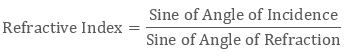Simple Application of Refraction

Let us consider a coin placed inside an empty container. We know that, we can see any object only when the light of the object reach our eyes. Thus, when we try to see the coin from the side of the container, it’s not visible to our eyes. But once the container is full of water, the light from the coin get refracted (denser to rarer medium) and reach our eyes. Thus, when we see the coin, it seems to be raised up from its actual position. This virtual position of coin is defined as apparent depth of the coin.Refractive Index (µ) of a liquid in the container can be defined as the ratio of actual depth of the coin to the apparent depth of the coin.

Another real life example of refraction of light in the atmosphere is the visibility of the sun before the actual sunrise and sunset. The refractive index of air w.r.t vacuum is 1. Due to this the direction of the sun is apparently shifted by half degree. Thus the time difference between the actual sunset and apparent sunset is 2 minutes.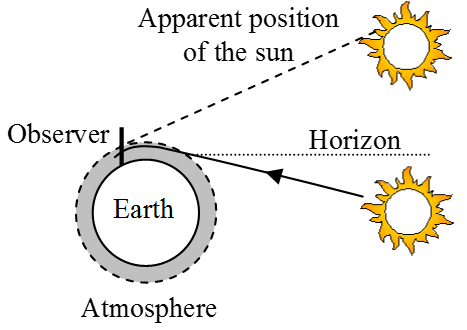Refraction through Lens

Before learning how refraction takes place in lens, let us first define what is Lens? A lens can be defines as a transparent medium with two spherical surface. They are also known as Spherical Lenses because of the fact that they are made from spheres.

Basically, there are two types of spherical lenses:

(a) Concave and (b) Convex Lens

Concave Lens

The concave lens is designed in such a way that the rim of the lens is made thicker and the rest of the portion is made thinner. Thus it has the property of spreading the light from a point. Due to this diverging property, a concave lens is also known as Diverging Lens.

Convex Lens

The convex lens is different from the concave lens in the way it is designed. Here, the rim is made thinner and the rest of the portion is made thicker. Thus the light travelling through the convex lens appear to converge at a point. Thus it is also known as the Converging Lens.

Image formation by Lenses

Few basic rules to be followed to determine the position and nature of the image formed by lenses.

A ray of light which is parallel to the principal axis, after getting refracted through the lenses appears to converge on the principal focus in case of convex lens. Whereas, in case of concave lens it appears to diverge off the principal focus.

A ray travelling through the optical centre of lens passes through the lens symmetrically and undeviated

A ray of light emerges parallel to the principal axis, after it passes through the first principal focus F.

Image formation by Convex Lens

Following the above rules let us find the position and nature of image, when the objects are placed at different positions:

Case a: When the object is placed between the optical centre O and focus F.The image formed is virtual, upright and magnified.

Case b: When the object is placed at the first focus F.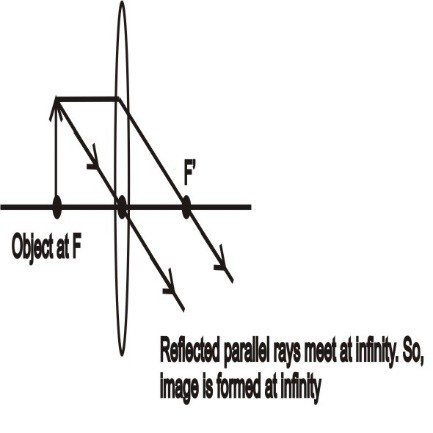The image is formed at infinity. The nature of the image formed is real and very much magnified

Similarly one can try forming the images of objects at various positions by following the basic rules of image formation in spherical lenses.

Image formation by Concave Lens

In case of concave lens, whatever may be the position of the object, the nature of the image formed is always small, virtual and erect. Also the image is formed only between focus and optical centre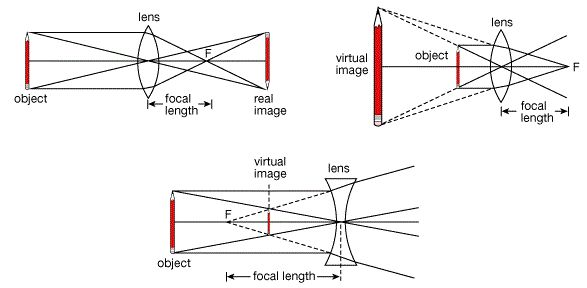Sign convention in Spherical Lenses

All distances in the lens are measured from the optical centre.

Distances that are measured in the direction of the incident ray is considered to be positive and negative if measured in the opposite direction.

The height of the object is taken to be positive if measured above principal axis and negative if measured below principal axis.

Formula of Lens

Considering the above sign convention for lenses, the relationship between object distance (u), image distance (v) and focal length (f) of a lens can be given as:Focal length of concave lens is taken as negative whereas for convex lens it is taken as positive.

Magnification of Lens

Magnification of lens can be defined as the ratio of image size to that of the object size.

It is given as,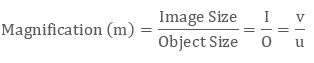It the value of ‘m’ is positive, then the image formed is erect. Similarly, if the value of ‘m’ is negative, then an inverted image is formed.

Power of a Lens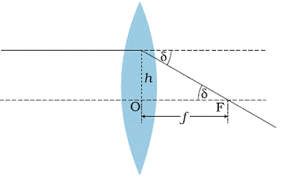The power of lens can be defined as the tan of the angle by which a beam of light converges or diverges from optical centre at unit point.If we consider height = 1, then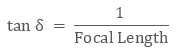Thus,The power of lens is considered positive for concave lens and negative for convex lens. The S.I. unit for power of a lens is given by D (dioptre).

Combination of thin Lenses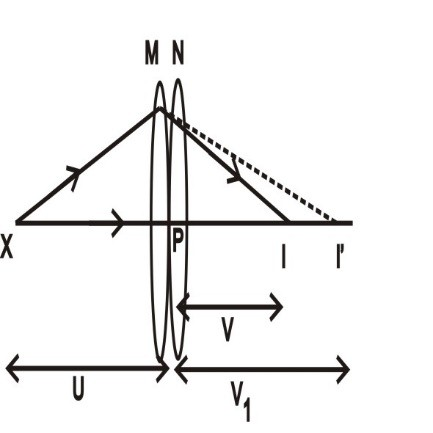Let us place two thin lenses M and N each having a focal length of f1 and f2 in contact with each other. Let the object be placed at point X which is beyond the focus of lens M.  Since the lenses are thin, therefore we consider their optical centre to be coincident at point P. The rays emanating from first lens M, modifies itself after considering the angle at which the rays strike the second lenses. For our convenience, we presume the image formed by the first lens M in the above diagram. The presumed image is formed at I’ which serves as the virtual image for the second lens.

The image formed by first lens can be given as: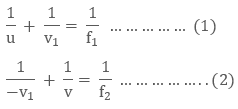Adding equation (1) and (2) we get,Now if we consider the two lens to have a common focal length f, we get,Comparing equation (3) and (4) we get,The power of lens (P) can also be defined as the reciprocal of focal length. Thus the above equation can be replaced as,

P = P1 + P2

Thus, it can be concluded that the power of thin lenses in contact is equal to the algebraic sum of the power of individual lenses.

Application of Spherical Lenses

Convex Lens:

Used in camera lenses

Used in microscopes, telescopes etc.

Used to detect hypermetropia or long sightedness

Concave Lens:

Used for detecting or correcting Myopia

Used as flashlights

Q1. What is the power of a lens?

Sol. The power of a lens can be defined as the Reciprocal of Focal Length. It can also be defines as the power of the lens to converge or diverge parallel beam of lights.

Q2. What is first focus and second focus in a spherical lens?

Sol. Since the lens have two curved surface, therefore each surface has their own foci. This two foci is termed as first focus and second focus of the lens

Q3. What is the importance of magnification?

Sol. Magnification helps us to determine the nature, position and size of the image. If magnification is negative then the image formed is real and inverted. If the magnification is positive, then the image formed is virtual and erect.

Watch this Video for more reference

Refraction
```### Course Features

• 101 Video Lectures
• Revision Notes
• Previous Year Papers
• Mind Map
• Study Planner
• NCERT Solutions
• Discussion Forum
• Test paper with Video Solution